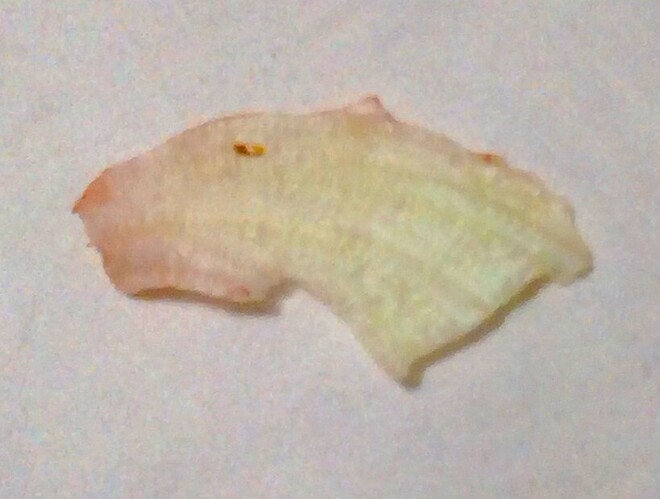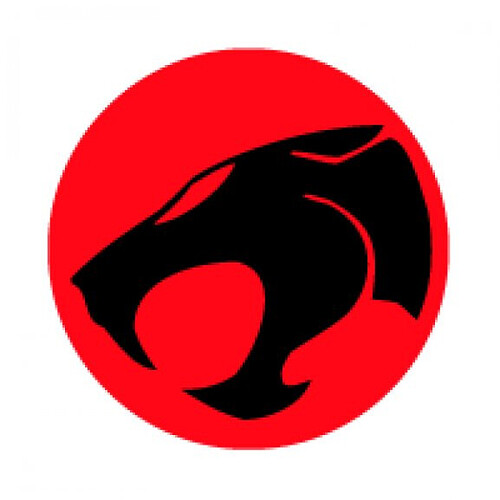-=+=- -=+=- -=+=- -=+=- -=+=- -=+=- -=+=- -=+=- -=+=- -=+=- -=+=- -=+=- -=+=- -=+=- -=+=- -=+=- -=+=- -=+=- -=+=- -=+=- -=+=- -=+=- -=+=- -=+=- -=+=- -=+=- -=+=- -=+=- -=+=- -=+=- (c) WidthPadding Industries 1987 0|199|0 -=+=- -=+=- -=+=- -=+=- -=+=- -=+=- -=+=- -=+=- -=+=- -=+=- -=+=- -=+=- -=+=- -=+=- -=+=- -=+=- -=+=- -=+=- -=+=- -=+=- -=+=- -=+=- -=+=- -=+=- -=+=- -=+=- -=+=- -=+=- -=+=- -=+=-
Socoder -> Killer Bites -> Food that looks like stuff

Posted : Sunday, 17 October 2021, 23:12
ProHiScore### Food that looks like stuff

I found a piece of lettuce, that looks like a panther.....|update| -=-=- |update|Posted : Monday, 18 October 2021, 02:57
spinalI'd say more like a Capybara than a Panther...

-=-=-
Check out my excellent homepage!Posted : Monday, 18 October 2021, 08:12
ProHiScoreYeah, now that you point that out. But those aren't as fresh in my mind as Thundercats.

I've heard those make good pets.Pumped up rats